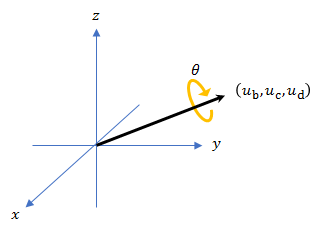dist

Description

example

distance = dist(quatA,quatB) returns the angular distance in radians between two quaternions, quatA and quatB.

Examples

collapse all

Calculate the quaternion distance between a single quaternion and each element of a vector of quaternions. Define the quaternions using Euler angles.

q = quaternion([0,0,0],'eulerd','zyx','frame')
q = quaternion
1 + 0i + 0j + 0k

qArray = quaternion([0,45,0;0,90,0;0,180,0;0,-90,0;0,-45,0],'eulerd','zyx','frame')
qArray = 5x1 quaternion array
0.92388 +         0i +   0.38268j +         0k
0.70711 +         0i +   0.70711j +         0k
6.1232e-17 +         0i +         1j +         0k
0.70711 +         0i -   0.70711j +         0k
0.92388 +         0i -   0.38268j +         0k

quaternionDistance = 5×1

45.0000
90.0000
180.0000
90.0000
45.0000

If both arguments to dist are vectors, the quaternion distance is calculated between corresponding elements. Calculate the quaternion distance between two quaternion vectors.

angles1 = [30,0,15; ...
30,5,15; ...
30,10,15; ...
30,15,15];
angles2 = [30,6,15; ...
31,11,15; ...
30,16,14; ...
30.5,21,15.5];

qVector1 = quaternion(angles1,'eulerd','zyx','frame');
qVector2 = quaternion(angles2,'eulerd','zyx','frame');

ans = 4×1

6.0000
6.0827
6.0827
6.0287

Note that a quaternion represents the same rotation as its negative. Calculate a quaternion and its negative.

qPositive = quaternion([30,45,-60],'eulerd','zyx','frame')
qPositive = quaternion
0.72332 - 0.53198i + 0.20056j +  0.3919k

qNegative = -qPositive
qNegative = quaternion
-0.72332 + 0.53198i - 0.20056j -  0.3919k

Find the distance between the quaternion and its negative.

dist(qPositive,qNegative)
ans = 0

The components of a quaternion may look different from the components of its negative, but both expressions represent the same rotation.

Input Arguments

collapse all

Quaternions to calculate distance between, specified as comma-separated quaternions or arrays of quaternions. quatA and quatB must have compatible sizes:

• size(quatA) == size(quatB), or

• numel(quatA) == 1, or

• numel(quatB) == 1, or

If one of the quaternion arguments contains only one quaternion, then this function returns the distances between that quaternion and every quaternion in the other argument.

Data Types: quaternion

Output Arguments

collapse all

Angular distance in radians, returned as an array. The dimensions are the maximum of the union of size(quatA) and size(quatB).

Data Types: single | double

Algorithms

The dist function returns the angular distance between two quaternions.

A quaternion may be defined by an axis (ub,uc,ud) and angle of rotation θq: $q=\mathrm{cos}\left({\theta }_{q}}{2}\right)+\mathrm{sin}\left({\theta }_{q}}{2}\right)\left({u}_{b}\text{i}+{u}_{c}\text{j}+{u}_{d}\text{k}\right)$.Given a quaternion in the form, $q=a+b\text{i}+c\text{j}+d\text{k}$, where a is the real part, you can solve for the angle of q as ${\theta }_{q}=2{\mathrm{cos}}^{-1}\left(a\right)$.

Consider two quaternions, p and q, and the product $z=p*\text{conjugate}\left(q\right)$. As p approaches q, the angle of z goes to 0, and z approaches the unit quaternion.

The angular distance between two quaternions can be expressed as ${\theta }_{\text{z}}=2{\mathrm{cos}}^{-1}\left(\text{real}\left(z\right)\right)$.

Using the quaternion data type syntax, the angular distance is calculated as:

angularDistance = 2*acos(abs(parts(p*conj(q))));